Next: Wave Propagation in Magnetized Up: Wave Propagation in Uniform Previous: Polarization of Electromagnetic Waves

The electromagnetic force acting on an electron is given by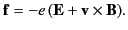(817)

If the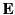and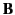fields in question are due to an electromagnetic wave propagating through a dielectric medium then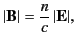(818)

where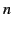is the refractive index. It follows that the ratio of the magnetic to the electric forces acting on the electron is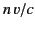. In other words, the magnetic force is completely negligible unless the wave amplitude is sufficiently high that the electron moves relativistically in response to the wave. This state of affairs is rare, but can occur when intense laser beams are made to propagate through plasmas.

Suppose, however, that the dielectric medium contains an externally generated magnetic field,. This can easily be made much stronger than the optical magnetic field. In this case, it is possible for a magnetic field to affect the propagation of low amplitude electromagnetic waves. The electron equation of motion (779) generalizes to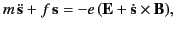(819)

where any damping of the motion has been neglected. Letbe directed in the positive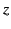-direction, and let the wave propagate in the same direction. These assumptions imply that theand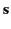vectors lie in the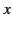-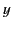plane. The previous equation reduces to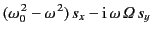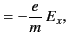(820)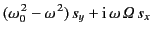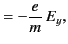(821)

provided that all perturbed quantities have an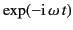time dependence. Here,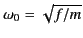, and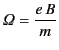(822)

is the electron cyclotron frequency. Let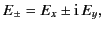(823)

and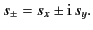(824)

Note that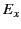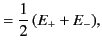(825)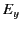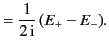(826)

Equations (821) and (822) reduce to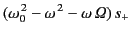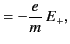(827)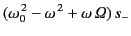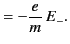(828)

Defining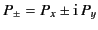, it follows from Equation (778) that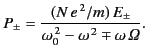(829)

Finally, from Equation (784), we can write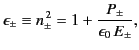(830)

giving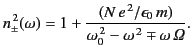(831)

According to the dispersion relation (832), the refractive index of a magnetized dielectric medium can take one of two possible values, which presumably correspond to two different types of wave propagating parallel to the-axis. The first wave has the refractive index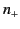, and an associated electric field [see Equations (826) and (827)]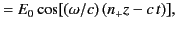(832)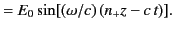(833)

This corresponds to a left-hand circularly polarized wave propagating in the-direction at the phase velocity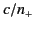. The second wave has the refractive index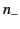, and an associated electric field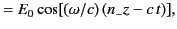(834)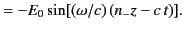(835)

This corresponds to a right-hand circularly polarized wave propagating in the-direction at the phase velocity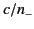. It is clear from Equation (832) that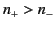. We conclude that, in the presence of a-directed magnetic field, a-directed left-hand circularly polarized wave propagates at a phase velocity that is slightly less than that of the corresponding right-hand wave. It should be remarked that the refractive index is always real (in the absence of damping), so the magnetic field gives rise to no net absorption of electromagnetic radiation. This is not surprising because a magnetic field does no work on charged particles, and cannot therefore transfer energy from a wave propagating through a dielectric medium to the medium's constituent particles.

We have seen that right-hand and left-hand circularly polarized waves propagate through a magnetized dielectric medium at slightly different phase velocities. What does this imply for the propagation of a plane polarized wave? Let us add the left-hand wave whose electric field is given by Equations (833) and (834) to the right-hand wave whose electric field is given by Equations (835) and (836). In the absence of a magnetic field,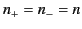, and we obtain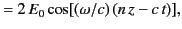(836)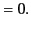(837)

This, of course, corresponds to a plane wave (polarized along the-direction) propagating along the-axis at the phase velocity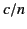. In the presence of a magnetic field, we obtain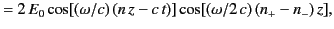(838)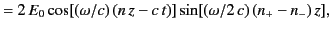(839)

where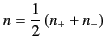(840)

is the mean index of refraction. Equations (839) and (840) describe a plane wave whose angle of polarization with respect to the-axis,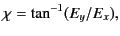(841)

rotates as the wave propagates along the-axis at the phase velocity. In fact, the angle of polarization is given by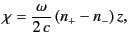(842)

which clearly increases linearly with the distance traveled by the wave parallel to the magnetic field. This rotation of the plane of polarization of a linearly polarized wave propagating through a magnetized dielectric medium is known as Faraday rotation (because it was discovered by Michael Faraday in 1845).

Assuming that the cyclotron frequency,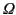, is relatively small compared to the wave frequency,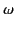, and also thatdoes not lie close to the resonant frequency,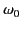, it is easily demonstrated that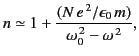(843)

and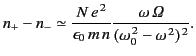(844)

It follows that the rate at which the plane of polarization of an electromagnetic wave rotates as the distance traveled by the wave increases is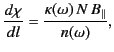(845)

where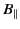is the component of the magnetic field along the direction of propagation of the wave, and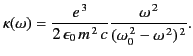(846)

If the medium in question is a tenuous plasma then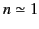, and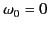. Thus,(847)

In this case, the rate at which the plane of polarization rotates is proportional to the product of the electron number density and the parallel magnetic field-strength. Moreover, the plane of rotation rotates faster for low frequency waves than for high frequency waves. The total angle by which the plane of polarization is twisted after passing through a magnetized plasma is given by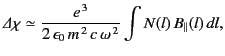(848)

assuming that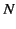andvary on length-scales that are large compared to the wavelength of the radiation. This formula is regularly employed in radio astronomy to infer the magnetic field-strength in interstellar space.Next: Wave Propagation in Magnetized Up: Wave Propagation in Uniform Previous: Polarization of Electromagnetic Waves
Richard Fitzpatrick 2014-06-27